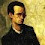Ir al contenido principal

## Due April 24th

### Problem 1

Let $U,V\subset\mathbb C$ open, $f:U\to V$ holomorphic and $u:V\to\mathbb C$ harmonic. The $u\circ f$ is harmonic in $U$.

### Problem 2

Let $f\in C(\partial \mathbb D)$ and $\psi\in\text{Aut}(\mathbb D)$. Then $\mathscr P(f\circ\psi) = \mathscr Pf\circ\psi$. (A sketch of the proof of this result is given in the text --Theorem 10.2.0--; give the details.)

### Problem 3

Explain why the following "proof" that there is no continuous function on $\bar{\mathbb D}$, holomorphic in $\mathbb D$ and equal to $f(e^{it}) = e^{-it}$ on the boundary, is wrong:
Let $u\in C(\bar{\mathbb D})$ be holomorphic in $\mathbb D$ with $u|_{\partial\mathbb D} = f$. Then $v(z) = u(z) - 1/z$ is holomorphic in $\mathbb D\setminus\{0\}$, vanishing on the boundary of $\mathbb D$. Since the set $\partial\mathbb D$ has an accumulation point and $\mathbb D\setminus\{0\}$ is connected, then $v$ vanishes everywhere. Thus $u(z) = 1/z$, a contradiction.

### Problem 4

Explain why the following "proof" of $\mathscr C f = \mathscr P f$, for $f\in C(\partial \mathbb D)$, is incorrect:
Since $\mathscr C f$ is holomorphic, then it is harmonic. Since $\mathscr P f$ is the only harmonic function in the disk with boundary values given by $f$, then $\mathscr C f$ must be equal to $\mathscr P f$.

### Comentarios

1.En el problema 1, ¿u es "real-valued"?

1.No necesariamente